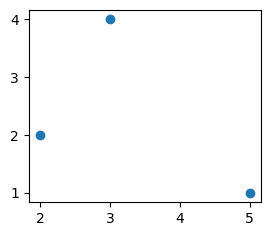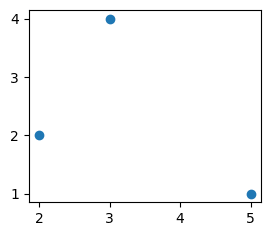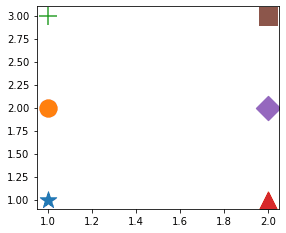search
Search
Map of Data Science
Guest 0reps
exit_to_appLog out
Map of data science
Thanks for the thanks!
close
account_circle
Profile
exit_to_app
Sign out
search
keyboard_voice
close
Searching Tips
Search for a recipe:
"Creating a table in MySQL"
Search for an API documentation: "@append"
Search for code: "!dataframe"
Apply a tag filter: "#python"
Useful Shortcuts
/ to open search panel
Esc to close search panel
to navigate between search results
d to clear all current filters
Enter to expand content previewDoc SearchCode Search BetaSORRY NOTHING FOUND!
mic
Start speaking...Voice search is only supported in Safari and Chrome.
Shrink
Navigate to
A
A
brightness_medium
share
arrow_backShareTwitterFacebook
check_circle
Mark as learned
thumb_up
0
thumb_down
0
chat_bubble_outline
0
auto_stories new
settings

# Drawing a scatterplot in Matplotlib

Matplotlib
chevron_right
Cookbooks
chevron_right
Graphs Cookbook
schedule Jul 1, 2022
Last updated
local_offer PythonMatplotlib
Tags
map
Check out the interactive map of data science

# Using the scatter function

To draw a basic 2D scatter plot in Matplotlib, we could use the `scatter(~)` function like so:

``` fig, ax = plt.subplots()ax.scatter([5,2,3], [1,2,4])plt.show() ```

The first argument is an array of your `x`s, and the second is an array of your `y`s.

This produces the following:# Using the plot function

To draw a basic 2D scatter plot in Matplotlib, we could also use the `plot(~)` function like so:

``` plt.plot([5,2,3], [1,2,4], "o")plt.show() ```

Here, the third argument `"o"` indicates that you don't want the data points to be connected. This is needed since the default behavior is to connect the dots.

This produces the following:# Customizing our plots

Both the `scatter(~)` and `plot(~)` methods allow for flexible customization.

## Marker size

Pass in the `markersize` parameter for `plot(~)` and the `s` parameter for `scatter(~)`:

``` plt.scatter([3,4],[5,6], s=10)plt.plot([3,4],[5,6], markersize=10) ```

## Marker color

Pass in the `color` parameter for both `plot(~)` and `scatter(~)`:

``` plt.plot([3,4], [5,6], color="r")plt.plot([3,4], [5,6], color="red")plt.plot([3,4], [5,6], color="#FF0000")plt.plot([3,4], [5,6], color=(1,0,0)) # Warning: this isn't your typical (0-255) interval ```

## Marker style

Pass in the `marker` parameter for both `plot(~)` and `scatter(~)`:

``` plt.scatter(, , marker="*", s=300) # starplt.scatter(, , marker="o", s=300) # circleplt.scatter(, , marker="+", s=300) # plusplt.scatter(, , marker="^", s=300) # triangleplt.scatter(, , marker="D", s=300) # diamondplt.scatter(, , marker="s", s=300) # square ```

This produces the following:The colors are automatically chosen by Matplotlib.

# Difference between functions scatter and plot

Both functions can be used to draw scatter plots, but the difference is that the `plot(~)` function is more efficient but less flexible than the `scatter(~) `function. The `plot(~)` function does not allow for customization of individual points (e.g. changing color and size), while the `scatter(~)` allows for this. The implication of such a difference is that the `plot(~)` function has far less jobs to handle, making it more efficient.

As a rule of thumb, if you do not need to customize individual points, then opt for the `plot(~)` function.